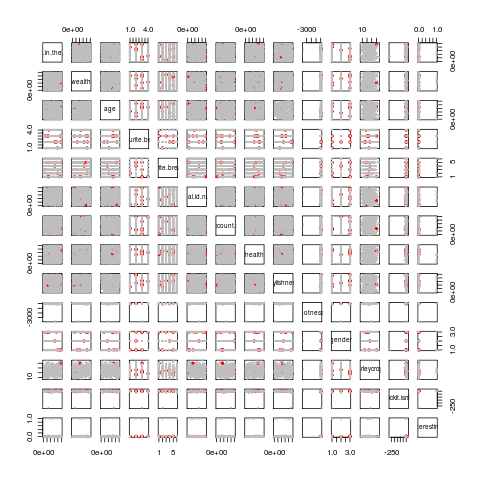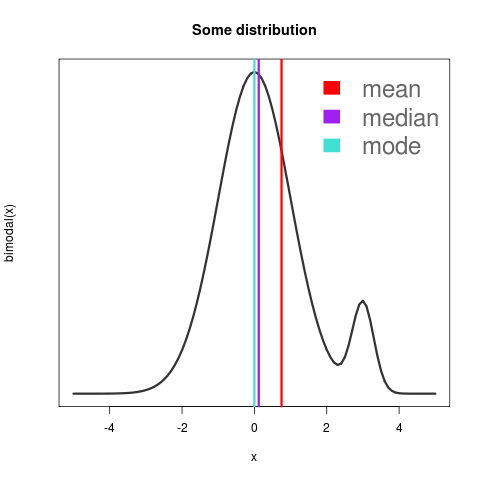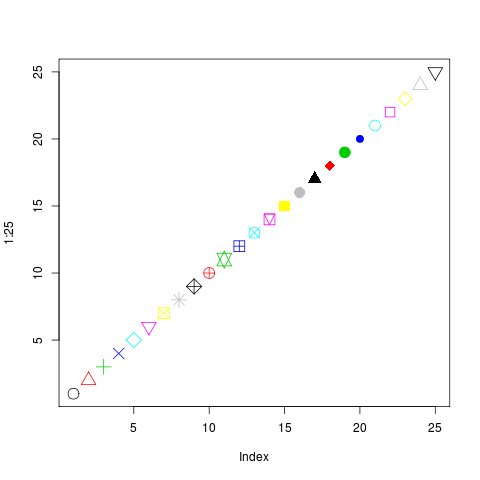Want to share your content on R-bloggers? click here if you have a blog, or here if you don't.

If you’re using `base::plot` in `R` for the first time (for example if you do `plot(pima)` or `plot(faithful)` (use `??pima` if you can’t find the dataset)) you may have looked at `?plot` (2 page help file) or `?par` (12 page help file) to figure out what’s going on.

`> plot(faithful, pch=19, col=rgb(.1,.1,.1,.5), cex=.6)`Firstly: what is `par`? When you type `par( lwd=3, col="#333333", yaxt="n")`, it will open an empty box that will hold your next `plot( dnorm, -3, 3)`. You can run different plots in the box and as long as you don’t close it, the line-width will be 3 times bigger than default, and the colour will be dark-grey.

There are a lot of plotting options. Here are the ones I use regularly:

• `cex = .8`. Decreases the size of type or plotted points by 20%.
• `par(new=TRUE)`. Use this to plot two things on top of each other. Beware, the labels will overprint over each other too (but this doesn’t matter for quick, casual plots).• `col = "red"`, `col = "#333333"`. I think #333333 is the best default colour and I use red if a point or line needs to stand out.• `col=rgb(.1,.1,.1,.5)`. This is another decent grey for overplotting. I used this in the Old Faithful plot above. The first three numbers are Red, Green, Blue and the fourth is Transparency.
• `lwd = 3`. This is a good line width, I think, especially with the dark grey col=”#333333”.
• `pch = 19`. Plots points with a small circle. `pch=20` is a slightly larger dot and `pch=15` is a square. Read after the second group of bullets for more info.
• `png("name of the plot.png")`. Then do `plot(x)`, `par(new=TRUE)`, `plot(y)`, `par(new=TRUE)`, `plot(z)`, and remember to finish it off with `dev.off()`. [`dev.off()` means device off; the `par()` window and the `png()` file are considered “graphic devices”.]Here are the ones I use less regularly, but still more than weird stuff like oma, mex, mai, etc.

• `lend = butt`. Line ending is square rather than mitred. I use this before I make a histogram.
• `ylog=TRUE`, `xlog=TRUE`. “Hubble made this significantly worse chart before it was discovered that all data look like straight lines on log-log plots.” —Lawrence Krauss
• `las=1`. If you want all of your axis labels to be printed horizontally.
• `mfrow=c(2,2)`. If you want to juxtapose four plots next to each other. mfrow and they will write like a typewriter, left-to-right and starting over on a new line after 2 spots have been filled in. mfcol=c(3,3) and they will fill in vertically. (Try it if what I said doesn’t make sense.)
• yaxs=”n”. This suppresses printing the vertical axis labels. I do this when plotting a distribution because the vertical numbers aren’t meaningful.
• `main="It's a plot about nothing. Don't you get it? People _love_ nothing!"`. This is the title of the plot.
• `legend( "top right", legend=c("control", "placebo", "test group"), fill = c("black", "#333333", "red"), border="white", bty="n")`. This is how I find legends look good. You should only need to change the placement, legend, and fill to make it work for your plot.
• One more awesome  advice from the StackOverflow `R` community: how to get some sweet, sweet log-axis tickmarks. Read all about it.

Most of these can be done inside of `plot( dpois, 0, 15, lwd = 3)` or beforehand in a `par(lwd=3); plot( dpois, 0, 15)`. With `par(new=TRUE)` and par(mfrow=c(2,2)), though, you need to do them in a `par()` beforehand.

If you forget what the colours or the pch shapes are, do this: `plot( 1:25, pch=1:25, col=1:25)`. You’ll get this:So basically, you only want `pch=19` and sometimes `pch=20` or `pch=15`, like I said.

One more thing you might like to learn is how to colour important data points red and normal ones grey. See this.